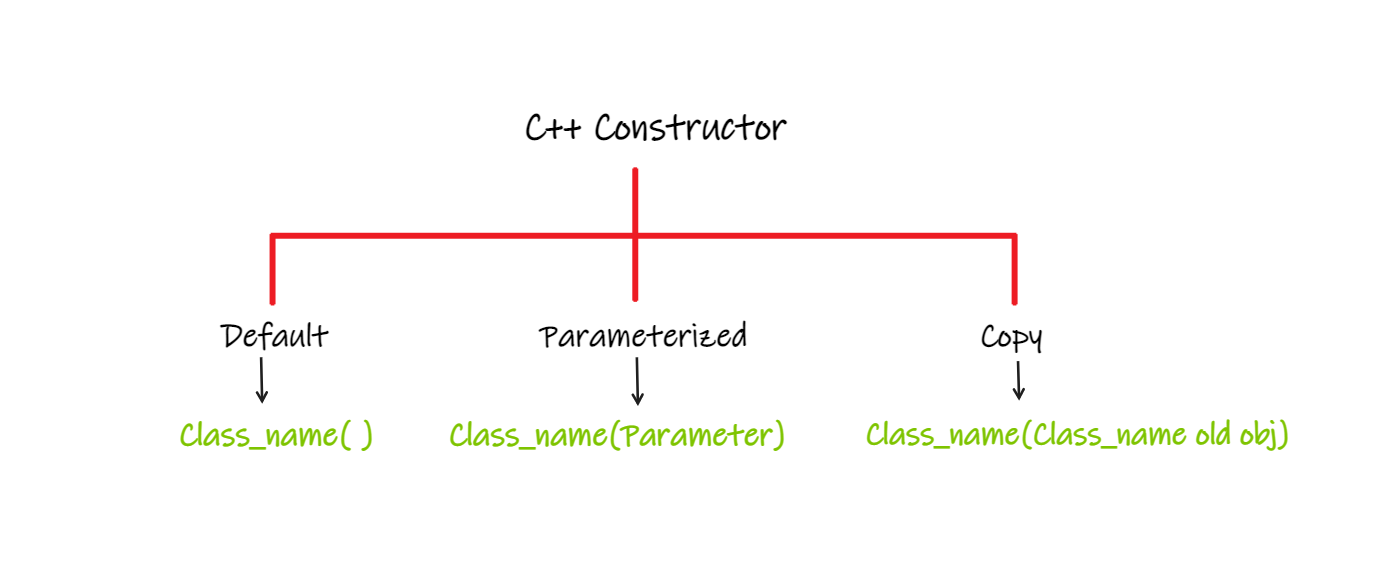# Constructor in C++

### What is Constructor in C++?

A constructor in C++ is a special method that is automatically called when an object of a class is created.

In class-based object-oriented programming, The constructor is a special method that is used to initialize a newly created object and is called just after the memory is allocated for the object.

If no user-defined constructor is provided for a class, the compiler initializes member variables to their default values.

Constructors can also take parameters (just like regular functions).

A constructor is a special member function of the class because it does not have any return type. Constructor has the same name as the name of a class

### Types of Constructors:In C++ there are three types of constructors namely

• Default constructor: A constructor without any arguments or with the default value for every argument is said to be the default constructor. They are used to create objects, which do not have any specific initial value.  If no constructors are explicitly declared in the class, a default constructor is provided automatically.

Code:

## C++ Code

``````#include <bits/stdc++.h>

using namespace std;

class construct {
public:
int x, y;

// Default Constructor with default values
construct() {
x = 5;
y = 10;
}
};

int main() {
// Default constructor called automatically
// whenever the object is created
construct z;
cout << "x is: " << z.x << "\n" << "y is: " << z.y;
return 0;
}``````

Output:

x is: 5
y is: 10

• Parameterized constructor: A constructor which takes arguments as input is called a parameterized constructor. It is used to provide different values to distinct objects. When we call the constructor (by creating an object of the class), we pass parameters to the constructor, which will set the value of the corresponding attributes to the same

Code:

## C++ Code

``````#include <bits/stdc++.h>

using namespace std;

class values {
private:
int a, b;

public:
// Parameterized Constructor
values(int x, int y) {
a = x;
b = y;
}

int getA() {
return a;
}
int getB() {
return b;
}
};

int main() {
// Constructor called
values v(5, 10);

// values assigned by constructor
cout << "v1.x = " << v.getA() << "\n" << "v2.y = " << v.getB();

return 0;
}``````

Output:

v1.x = 5
v2.y = 10

• Copy constructor: A copy constructor is a member function that initializes an object using another object of the same class. A copy constructor initializes an object by copying the member values from an object of the same type. If your class members are all simple types such as normal values, the compiler-generated copy constructor is sufficient and you don’t need to define your own.

Code:

## C++ Code

``````#include<iostream>

using namespace std;

class Point {
private:
int x, y;
public:
Point(int x1, int y1) {
x = x1;
y = y1;
}

// Copy constructor
Point(const Point & p1) {
x = p1.x;
y = p1.y;
}

int getX() {
return x;
}
int getY() {
return y;
}
};

int main() {
Point p1(10, 15); // Normal constructor is called here
Point p2 = p1; // Copy constructor is called here

// Let us access values assigned by constructors
cout << "p1.x = " << p1.getX() << ", p1.y = " << p1.getY();
cout << "\np2.x = " << p2.getX() << ", p2.y = " << p2.getY();

return 0;
}``````

Output:

p1.x = 10, p1.y = 15
p2.x = 10, p2.y = 15

Note: The constructor has the same name as the class, it is always public, and it does not have any return value.

Special thanks to Abhishek Yadav for contributing to this article on takeUforward. If you also wish to share your knowledge with the takeUforward fam, please check out this article